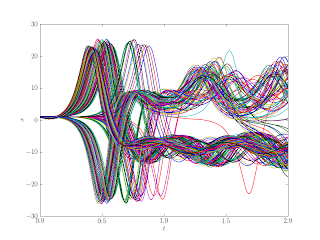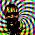## Monday, January 18, 2010

### Easy Full-Factorial Ensemble Experiments

This is another installment of the Lorenz63 series. In this post I wanted to demonstrate an ’experiment’ on the Lorenz ’63 system. The basics of these sorts of computer experiments as they relate to climate modeling are covered at climateprediction.net.

The main factors underlying our experimental design are initial conditions, parameters and forcing. Since we followed Roache’s advice about making our code Verifiable with the method of manufactured solutions (MMS) the ability to specify forcings is already included. Python has some nice built-in functions in the itertools module that make doing a computational experiment really easy. First all of the arguments for the time integrator are defined as lists (the ones which aren’t part of the experiment only have one element, so they remain constant):

# factors in our experimental design (all of the inputs to the system
# integrator, some only have one level):
nt = 2000
savevery = 
nsaves = nt / savevery + 1
T = 2.0
# the first element of the array is the initial condition, the rest
# are over-written with the solution:
x = [1.05*sp.ones(nsaves,dtype=float,order=’F’),
0.95*sp.ones(nsaves,dtype=float,order=’F’)]
y = [-1.05*sp.ones(nsaves,dtype=float,order=’F’),
-0.95*sp.ones(nsaves,dtype=float,order=’F’)]
z = [-10.5*sp.ones(nsaves,dtype=float,order=’F’),
-9.5*sp.ones(nsaves,dtype=float,order=’F’)]
t = [sp.zeros(nsaves, dtype=float, order=’F’)]
Ra = [27.0, 28.0, 29.0]
Pr = [9.0, 10.0, 11.0]
b = [8.0/3.0 - 0.1, 8.0/3.0 + 0.1]
h = [T / float(nt)]
tol = [1e-14]
maxits = 
forcing = [0, 1]
bx = [0.0]
by = [-1.0]
bz = [-10.0]

Then a call to itertools.product() gives us the Cartesian Product of all of those lists, which is a full-factorial set of the inputs (2 2 2 3 3 2 2 = 288 treatments in this example).

# this gives us an iterator of every combination of our factor levels:
full_factorial = itertools.product(
x, y, z, t, Ra, Pr, b, h, tol, maxits, forcing, bx, by, bz, savevery)

Once we have the iterator defined, we can calculate all of the trajectories associated with the input treatments in the experiment:

# iterate over all of the treatments in our design, the iterator
# yields the arguments directly:
for args in full_factorial:
L63.time_loop(*args)
xresult.append(args.copy())
yresult.append(args.copy())
zresult.append(args.copy())
tresult.append(args.copy())

The resulting trajectories are shown in Figure 1. It’s pretty clear that realistic uncertainties in parameters and initial conditions lead quickly to pretty diffuse predictive distributions. Part of the challenge of attribution studies is determining the effect of each change in parameter or forcing. In realistic models, the number of ’factors’ to vary is quite large.(a) X component(b) Y component(c) Z component
 Figure 1: Trajectories for the Full-Factorial Experiment

With such a low-dimension system, and few parameters it is easy enough to run the whole full-factorial, smarter experimental designs are required for experiments based on large simulation runs. A good reference on this is Design and Analysis of Computer Experiments.

#### 1 comment:

1.I just recommended nearly this exact experimental strategy to a new guy in my lab:
1. Screening runs. The initial set of evaluations is designed to pick out basic structure in the model, such as identifying the important or active model-inputs, plus some indication of the nature of the model-response to these inputs (e.g. linear, quadratic, linear in the log). A maximin latin hypercube can be an effective choice. Where we have strong prior information about which inputs are important (often the case with climate models), we may use such a design on the less important model-inputs, and a more structured design in the subspace of active inputs, as described next.
2. Interactions. In climate models, we expect interactions between model-inputs to be important in determining the model-outputs. With a large number of model-inputs, we cannot expect to explore all possible interactions, even if we limit ourselves to two-way effects. Therefore, we explore interactions initially in the active inputs. This second set of evaluations could follow a standard experimental design such as a fractionated factorial, which allows us to identify low-order interactions (e.g. two- and three-way). Another option which combines stages (i) and (ii) is to generate a screening design, and then assign the probable active inputs to the best subset in the design, e.g. the D-optimal subset.
3. Sequential. After the first two stages, we should have enough evaluations to build a useful emulator. In the third stage, we can use this emulator to select further evaluations. The simplest approach is to put additional evaluations into regions of the model-input space for which the predictive uncertainty, i.e. Graphic, is currently high. Such evaluations will tend quite naturally to avoid the previous evaluations in X.

Inference in ensemble experiments